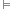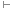# Symbolic Logic

Symbolic logic is the method of representing logical expressions through the use of symbols and variables, rather than in ordinary language. This has the benefit of removing the ambiguity that normally accompanies ordinary languages, such as English, and allows easier operation.

There are many systems of symbolic logic, such as classical propositional logic, first-order logic and modal logic. Each may have seperate symbols, or exclude the use of certain symbols.

## Logical Symbols

The following table presents several logical symbols, their name and meaning, and any relevant notes. The name of the symbol (under “meaning” links to a page explaining the symbol or term and its use). Note that different symbols have been used by different logicians and systems of logic. For the sake of clarity, this site consistently uses the symbols in the left column, while the “Notes” column may indicate other commonly-used symbols.

Symbol Meaning Notes
Operators (Connectives)
¬ negation (NOT) The tilde ( ˜ ) is also often used.
conjunction (AND) The ampersand ( & ) or dot ( · ) are also often used.
disjunction (OR) This is the inclusive disjunction, equivalent to and/or in English.
exclusive disjunction (XOR) ⊕ means that only one of the connected propositions is true, equivalent to either…or. Sometimes ⊻ is used.
| alternative denial (NAND) Means “not both”. Sometimes written as ↑
joint denial (NOR) Means “neither/nor”.
conditional (if/then) Many logicians use the symbol instead. This is also known as material implication.
biconditional (iff) Means “if and only if” ≡ is sometimes used, but this site reserves that symbol for equivalence.
Quantifiers
universal quantifier Means “for all”, so ∀xPx means that Px is true for every x.
existential quantifier Means “there exists”, so ∃xPx means that Px is true for at least one x.
Relations
implication α ⊨ β means that β follows from α
equivalence Also ⇔. Equivalence is two-way implication, so α ≡ β means αβ and βα.
provability Shows provable inference. αβ means that from α we can prove that β.
therefore Used to signify the conclusion of an argument. Usually taken to mean implication, but often used to present arguments in which the premises do not deductively imply the conclusion.
forces A relationship between possible worlds and sentences in modal logic.
Truth-Values
tautology May be used to replace any tautologous (always true) formula.
contradiction May be used to replace any contradictory (always false) formula. Sometimes “F” is used.
Parentheses
( ) parentheses Used to group expressions to show precedence of operations. Square brackets [ ] are sometimes used to clarify groupings.
Set Theory
membership Denotes membership in a set. If a ∈ Γ, then a is a member (or an element) of set Γ.
union Used to join sets. If S and T are sets of formula, S ∪ T is a set containing all members of both.
intersection The overlap between sets. If S and T are sets of formula, S ∩ T is a set containing those elemenets that are members of both.
subset A subset is a set containing some or all elements of another set.
proper subset A proper subset contains some, but not all, elements of another set.
= set equality Two sets are equal if they contain exactly the same elements.
absolute complement ∁(S) is the set of all things that are not in the set S. Sometimes written as C(S), S or SC.
- relative complement T - S is the set of all elements in T that are not also in S. Sometimes written as T \ S.
empty set The set containing no elements.
Modalities
necessarily Used only in modal logic systems. Sometimes expressed as [] where the symbol is unavailable.
possibly Used only in modal logic systems. Sometimes expressed as <> where the symbol is unavailable.

## Propositions, Variables and Non-Logical Symbols

The use of variables in logic varies depending on the system and the author of the logic being presented. However, some common uses have emerged. For the sake of clarity, this site will use the system defined below.

Symbol Meaning Notes
A, B, C … Z propositions Uppercase Roman letters signify individual propositions. For example, P may symbolize the proposition “Pat is ridiculous”. P and Q are traditionally used in most examples.
α, β, γ … ω formulae Lowercase Greek letters signify formulae, which may be themselves a proposition (P), a formula (P ∧ Q) or several connected formulae (φ ∧ ρ).
x, y, z variables Lowercase Roman letters towards the end of the alphabet are used to signify variables. In logical systems, these are usually coupled with a quantifier, ∀ or ∃, in order to signify some or all of some unspecified subject or object. By convention, these begin with x, but any other letter may be used if needed, so long as they are defined as a variable by a quantifier.
a, b, c, … z constants Lowercase Roman letters, when not assigned by a quantifier, signifiy a constant, usually a proper noun. For instance, the letter “j” may be used to signify “Jerry”. Constants are given a meaning before they are used in logical expressions.
Ax, Bx … Zx predicate symbols Uppercase Roman letters appear again to indicate predicate relationships between variables and/or constants, coupled with one or more variable places which may be filled by variables or constants. For instance, we may definite the relation “x is green” as Gx, and “x likes y” as Lxy. To differentiate them from propositions, they are often presented in italics, so while P may be a proposition, Px is a predicate relation for x. Predicate symbols are non-logical — they describe relations but have neither operational function nor truth value in themselves.
Γ, Δ, … Ω sets of formulae Uppercase Greek letters are used, by convention, to refer to sets of formulae. Γ is usually used to represent the first site, since it is the first that does not look like Roman letters. (For instance, the uppercase Alpha (Α) looks identical to the Roman letter “A”)
Γ, Δ, … Ω possible worlds In modal logic, uppercase greek letters are also used to represent possible worlds. Alternatively, an uppercase W with a subscript numeral is sometimes used, representing worlds as W0, W1, and so on.
{ } sets Curly brackets are generally used when detailing the contents of a set, such as a set of formulae, or a set of possible worlds in modal logic. For instance, Γ = { α, β, γ, δ }